﻿ Maths Olympiad (CMO) Previous Year Paper for KG

# CREST Mathematics Olympiad Class KG Previous Year Papers

CREST Mathematics Olympiad Previous Year Paper PDF for Class KG:

## Syllabus:

Section1: Basic Concepts on Comparison (Big-Small, Front-Back, Full-Empty, Heavy-Light, In-Out, More-Less, Right-Left, Same-Different, Tall-Short, Thick-Thin, Top-Bottom)
Numbers (Number names (1-50), Before, After, Between Numbers (1-20), Skip Counting (2 and 5))
Playing Numbers (1-digit addition and subtraction, Putting Signs like \'>\', \'<\' and \'=\')
Time (Days of a week, Months in a year)
Shapes (Square, Rectangle, Triangle, Circle, Oval, Sphere, Cone, Cube, Cylinder)

Achievers Section: Higher Order Thinking Questions - Syllabus as per Section 1

 Q.1 Complete the given series: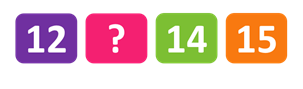Q.2 How many windows are shown in the picture?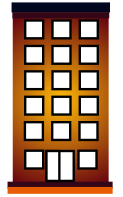Q.3 What is the name of the given shape?Q.4 Find the difference: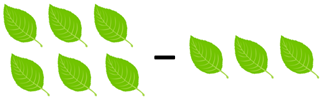Q.5 Which clock indicates 6:00?
 Q.6 Which number is given in the image?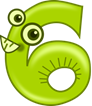Q.7 Which one is the back image of the given image: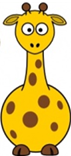Q.8 Complete the series: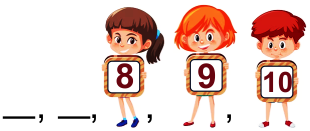Q.9 Add the numbers: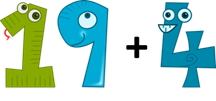Q.10 Find the chick which is different from others: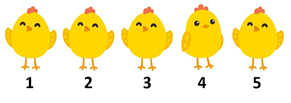Q.1 b Q.2 b Q.3 b Q.4 a Q.5 a Q.6 a Q.7 b Q.8 a Q.9 a Q.10 a

Students can download the CREST Mathematics Olympiad previous year paper pdf for class KG from this page. Each question in the paper carries 1 mark. The answer key for all the questions is also provided on the last page.

The CREST Mathematics Olympiad past year papers for class KG are a dependable resource to prepare for the CREST Mathematics Olympiad exam. By solving the CREST Mathematics Olympiad previous year paper, students can obtain an idea of the kinds of questions that will be asked in the exam. Also, practicing the previous year paper questions helps students to revise concepts quickly.